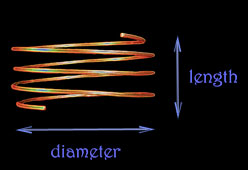Tools

These tools can be useful for finding various properties and dimensions of coils.

## Coil resonant frequency calculator

 This calculates a coil's resonant frequency (f), inductance (L), and self capacitance (C), given its length and diameter as input.

 Coil dimensions calculator     (quarter wave oscillator)This calculates the possible dimensions: lengths, diameters, etc., given the desired self resonant frequency (fr) of the coil as input. Assuming that the coil will be a quarter wave oscillator ( λ = 4 ℓw).

 Coil dimensions calculator     (half wave oscillator)This calculates the possible dimensions: lengths, diameters, etc., given the desired self resonant frequency (fr) of the coil as input. Assuming that the coil will be a half wave oscillator ( λ = 2 ℓw).

 This can be useful in obtaining the length of a wire on a spool. Entering the weight of the wire on the spool in ounces, and the wire's gauge, this will calculate the length of the wire on the spool.

 Calculates the necessary capacitance (C) for a tank circuit at a given resonant frequency (f), and inductance (L).

 Calculates the possible number of turns of wire for a primary coil, with the given input of the coils mass, diameter of the coil, and the wire's gauge.

 radiant arcs tesla coils magnetic field sensors self-oscillating voltage demo audio tests techstars application violet ray documents tools coil frequency calculator coil dimensions calculator wire length spool calculator capacitance calculator primary coil turns calculator home

back